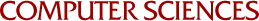## The Exact Solution of Systems of Linear Equations with Polynomial Coefficients

Michael T. McClellan
1971

Modular algorithms for linear equations solution, matrix inversion, determinant calculation, null space basis generation, and matrix multiplication are developed, all for matrices with polynomial entries. Theoretical computing times are obtained for all algorithms. The algorithms are programmed for Fortran IV, forming a module of the SAC-1 system for symbolic and algebraic calculation, and empirical computing times are given for representative cases.